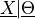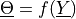# Create a conditional distribution¶

In this example we are going to build the distribution of the random vector X conditioned by the random variable Thetawith Theta obtained with the random variable Y through a function f```import openturns as ot
import openturns.viewer as viewer
from matplotlib import pylab as plt
```

create the Y distribution

```YDist = ot.Uniform(-1.0, 1.0)
```

create Theta=f(y)

```f = ot.SymbolicFunction(["y"], ["y", "1+y^2"])
```

create the X|Theta distribution

```XgivenThetaDist = ot.Uniform()
```

create the distribution

```XDist = ot.ConditionalDistribution(XgivenThetaDist, YDist, f)
XDist.setDescription(["X|Theta=f(y)"])
XDist
```

ConditionalDistribution(X with X|Theta~Uniform(Theta), Theta=f(Y), f=[y]->[y,1+y^2], Y~Uniform(a = -1, b = 1))

Get a sample

```XDist.getSample(5)
```
X|Theta=f(y) 1.431419 1.072163 0.362335 0.1600886 1.028835

draw PDF

```graph = XDist.drawPDF()
view = viewer.View(graph)
plt.show()
```